## Credit Card Fraud Detection With Classification Algorithms In PythonFraud transactions or fraudulent activities are significant issues in many industries like banking, insurance, etc. Especially for the banking industry, credit card fraud detection is a pressing issue to resolve.

These industries suffer too much due to fraudulent activities towards revenue growth and lose customer’s trust. So these companies need to find fraud transactions before it becomes a big problem for them.

Unlike the other machine learning problems, in credit card fraud detection the target class distribution is not equally distributed. It is popularly known as the class imbalance problem or unbalanced data issue.

Learn how to build machine learning models with classification algorithms to detect the credit card frauds in python

This makes this problem even more challenging to solve.

So In this article, we will explain to you how to build credit card fraud detection using different machine learning classification algorithms

Such as,

You will also get an idea about the impact of unbalanced data on the model’s performance.

Let us give you the list of contents that we will discuss in the next few minutes. Just to give you a glimpse about the topics that you are going to learn from this article.

Let’s begin the discussion by understanding why we need to find fraudulent transactions/activities in any industry.

## Why do we need to find fraud transactions?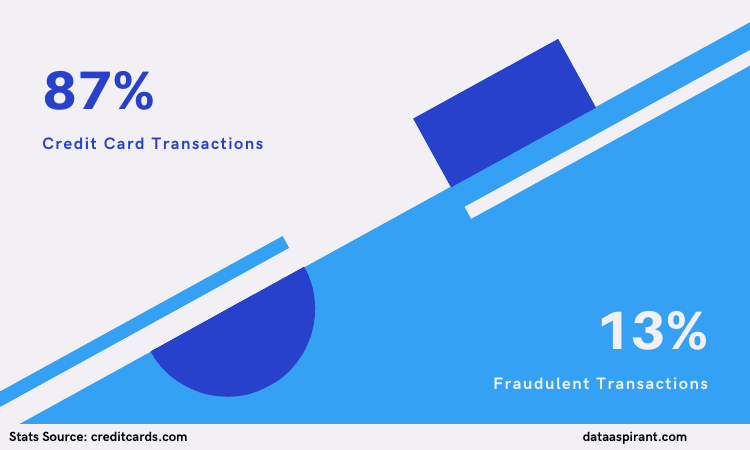Credit Card Fraudulent Transactions Percentanges

For many companies, fraud detection is a big problem because they find these fraudulent activities after they experience high loss

Fraud activities happen in all  industries. We can’t say only particular companies/industries suffer from these fraudulent activities or transactions.

But when it comes to financial-related companies, this fraud transaction becomes more of an issue/problem.  So these companies want to detect fraud transactions before the fraud activities turn into significant damage to their company.

In the current generation, with high-end technology, still, on every 100 credit card transactions, 13% are falling into the fraudulent activities reported by the creditcards website.

A survey paper mentioned that in the year 1997, 63% of companies experienced one fraud in the past two years, and in another year 1999, 57% of companies experienced at least one fraud in the last one year.

Here the point is not only fraud activities increase, but the way of doing scams also increases badly.

Companies suffer from detecting fraud, and due to these fraudulent activities, many companies worldwide have lost billions of dollars yearly.

And one more thing, for any company, customer’s trust is more important to achieve or reach some position in the business marketplace. If a company cannot find these fraudulent activities, companies lose customer’s trust; then, they will suffer from customer churn.

### Fraud Detection Approaches

So companies start to detect these fraud activities automatically by using smart technologies.

First, companies hire few people only for the detection of these kinds of activities or transactions. But here they must and should be experts in this field or domain, and also the team should have knowledge of how frauds occur in particular domains. This requires more resources, such as people’s effort and time.

Second, companies changed manual processes to rule-based solutions. But this one also fails most of the time to detect frauds.

Because in the real world, the way of doing frauds is changing drastically day by day. These rule-based systems follow some rules and conditions. If a new fraud process is different from others, then these systems fail. It requires adding that new rule to code and execute.

Now companies are trying to adopt Artificial Intelligence or machine learning algorithms to detect frauds. Machine learning algorithms performed very well for this type of problem.

## What is Credit Card Fraud Detection?Credit Card Fraud Detection

In the above section, we discussed the need for identifying fraudulent activities. The credit card fraud classification problem is used to find fraud transactions or fraudulent activities before they become a major problem to credit card companies.

It uses the combination of fraud and non-fraud transactions from the historical data with different people’s credit card transaction data to estimate fraud or non-fraud on credit card transactions.

In this article, we are using the popular credit card dataset. Let’s understand the data before we start building the fraud detection models.

## Understanding of Credit Card Dataset

For this credit card fraud classification problem, we are using the dataset which was downloaded from the Kaggle platform.

Before going to the model development part, we should have some knowledge about our dataset.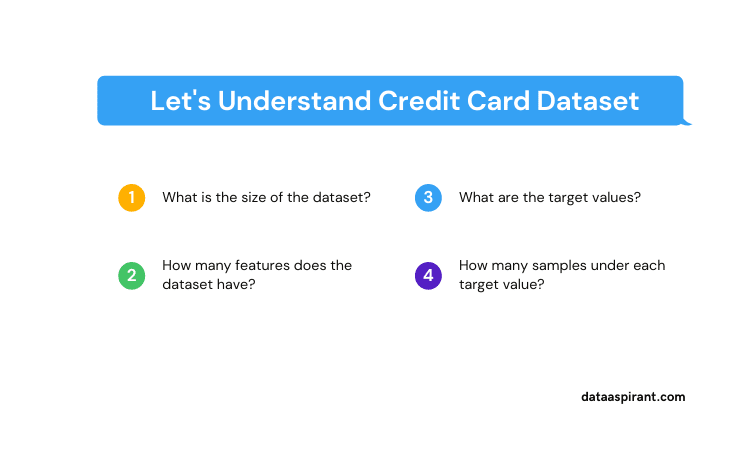Understand Credit Card Dataset

Such as

1. What is the size of the dataset?
2. How many features does the dataset have?
3. What are the target values?
4. How many samples under each target value? , etc.

If we know some information about the dataset, then we can decide what we have to do?.

What are the questions we discussed above, all  we can explore by using the python pandas library

### Data Explorations

First, we need to load the dataset. After downloading the dataset, extract the data and keep the file in the dataset under the project folder.

We can quickly load it using pandas.

Our dataset is a CSV(Comma Separated Values) file. We can use the read_csv function from pandas to read the file.

Ok, now find the answers for our above dataset related questions.

Dataset has 284807 rows and 31 features. The result of the shape variable is a tuple that has the number of rows, number of columns of the dataset.

We can see how the dataset looks like. The below command showcases  only five rows, head() by default, gives 5 samples.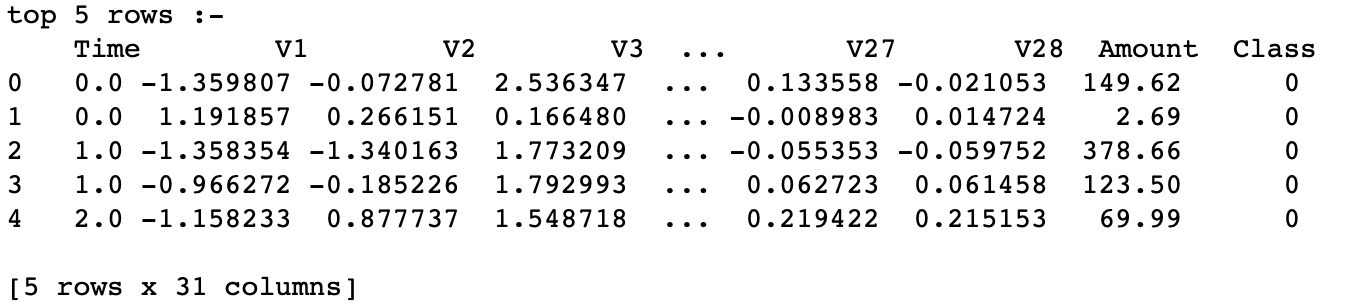Credit card data observations

If you want to see more samples from the top, pass the number representing the number of samples you want to see like fraud_df.head(10).

You can also see bottom samples by using the tail() function. Both are working in the same way.

We can get all the list of feature names.

From this, we know Class is the target variable, and the remaining all are features of our dataset.

Let’s see what are the unique values we are having for the target variable.

The target variable Class has 0 and 1 values. Here

• 0 for non-fraudulent transactions
• 1 for fraudulent transactions

Because we aim to find fraudulent transactions, the dataset’s target value has a positive value for that.

Still, What is pending in data exploration questions?

yeah, we have to check how many samples each target class is having.

Yeah, we have 284315 non-fraudulent transaction samples & 492 fraudulent transaction samples.

You are going to know the variation of this number of samples and how much impact on the model’s performance, how we can evaluate model performance for this data, etc.

Still, now you only know about the dataset, such

• Dataset size
• Number of samples(rows) and features(columns)
• Names of the features

Now we will discuss different data preprocessing techniques for our dataset.

The data preprocessing techniques will be completely different from the text preprocessing techniques we discussed in the natural language processing data preprocessing techniques article

## Credit Card Data Preprocessing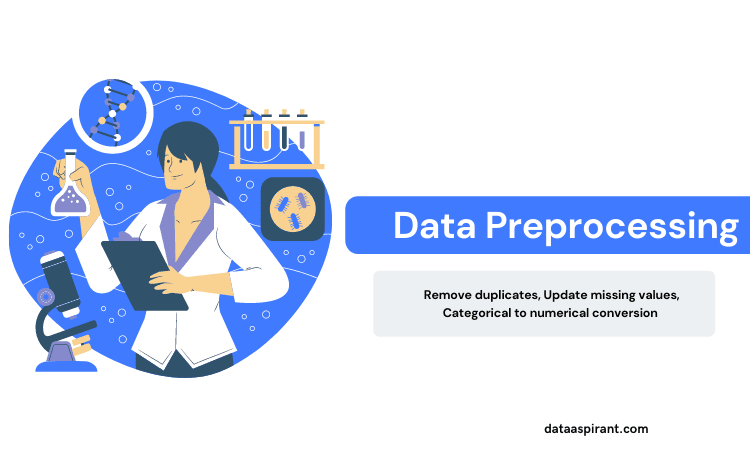Credit card data preprocessing

Preprocessing is the process of cleaning the dataset. In this step, we will apply different methods to clean the raw data to feed more meaningful data for the modeling phase. This method includes

• Remove duplicates or irrelevant samples
• Update missing values with the most relevant values
• Convert one data type to another example, categorical to integers, etc.

Okay, now we will spend a couple of minutes checking the dataset and applying corresponding techniques to clean data.

This step aims to improve the quality of the data.

### Removing irrelevant columns/features

In our dataset, only one irrelevant or not useful feature id Time. So we can drop that feature from the dataset.

If you want to drop more features from data, call drop() method with a list of feature names.

We can observe no feature name Time in the list of feature names after dropping the Time feature/column.

### Checking null or nan values

We can check the datatypes of all features and, at the same time, the number of non-null values of all features by using info() of pandas.

Null or nan values are nothing, but there is no value for that particular feature or attribute.

For example, these nan or null values are coming if the customer or user does not fill all information in the forms. Blank values are treated as null or nan values.

It’s okay; we can know all this information just by using info() from pandas.

See the result of dataset info();

it provides all information about our dataset, such as

• Total number of samples or rows
• Column names
• Number of non-null values
• The data type of each column

Our dataset doesn’t have any null values because the total number features are 284807 that ranges from 0-284806; all features have the same number of samples/rows.

### Data Transformation

Except for the Amount column, all column’s values are within some range of values. So let’s change the Amount columns values to a smaller range of numbers.

We can simply do this process by using StandardScaler from the sklearn library.

See the values of the Amount feature values are in high range compared to other feature values.

We will change values within a smaller range.

The scalar result is added as a new column with norm_amount name to the data frame after we drop the Amount column because there is no use with it.

### Splitting dataset

Now we will take all independent columns (target column is dependent and the remaining all are independent columns to each other), as X and the target variable as y.

Now we need to split the whole dataset into train and test dataset. Training data is used at the time of building the model and a test dataset is used to evaluate trained models.

By using the train_test_split method from the sklearn library we can do this process of splitting the dataset to train and test sets.

Now our dataset is ready for building models. Let’s jump to the development of  the model using machine learning algorithms such as decision tree and random forest classification algorithms from the sklearn module.

## Building Credit Card Fraud Detection using Machine Learning algorithms

Now we can build models using different machine learning algorithms. Before creating a model, we need to find the type of problem statement, which means is supervised or unsupervised algorithms.

Our problem statement falls under the supervised learning problem means the dataset has a target value for each row or sample in the dataset.

Supervised machine learning algorithms are two types

• Classification Algorithms
• Regression Algorithms

Our problem statement belongs to what type of algorithms?

Yeah, exactly.

Credit card fraud detection is a classification problem. Target variable values of Classification problems have integer(0,1) or categorical values(fraud, non-fraud). The target variable of our dataset ‘Class’ has only two labels – 0 (non-fraudulent) and 1 (fraudulent).

Before going further let us give an introduction for both decision tree classification and random forest classification. As in this article, we are going to use these two algorithms to build the credit card fraudulent activities identification model.

• Decision Tree Classification Algorithm
• Random Forest Classification Algorithm

### Decision Tree Algorithm Overview

The decision tree is the simplest and most popular classification algorithm. For building the model the decision tree algorithm considers all the provided features of the data and comes up with the important features.

Because of this advantage, the decision tree algorithms also used in identifying the importance of the feature metrics. Which used in handpicking the features.

Once the important features identified then the model trains with the training data to come up with a set of rules. These rules used in predicting future cases or for the test dataset.

This is a quick overview of the decision tree algorithm. If you want to learn more about the algorithm and implement in python, have a look at the below articles written by our team.

Now let’s see a quick overview of the random forest algorithm.

### Random Forest Algorithm Overview

The random forest algorithm falls under the ensemble learning algorithm category. In the random forest algorithm, we build N decision tree models.

All the models predict the target value. Using the majority voting approach the final target value will be predicted.

For building the individual decision tree, the random forest algorithm randomly creates the sample dataset. These sample datasets are called as the bootstrap samples.

Suppose we want to build the N decision trees to create the forest, the algorithm first creates N bootstrap samples. Later for each bootstrap sample, one decision tree model will build.

This is a quick overview of the random forest algorithm, If you want to learn more, please have a look at the below articles.

Now let’s go to the implementation part, the crazy one 🙂

## Credit Card Fraud Detection with Decision Tree AlgorithmCredit card fraud detection with decision tree

We will use the DecisionTreeClassifier class from the sklearn library to train and evaluate models. We use X_train and y_train data for training purposes. X_train is a training dataset with features, and y_train is the target label.

### Decision tree algorithm Implementation using python sklearn library

The output for the above code listed below.

Wow, our decision tree classification gives 99% accuracy on test data.

But why f1-score on label 1 too less ?.

Remember this point; we will discuss these metrics performances in the coming section of this article where we address the question

Why the accuracy evaluation metric is not suitable for this problem?

## Credit Card Fraud Detection with Random Forest AlgorithmCredit card fraud detection with random forest

Same as the above decision tree implementation, we use X_train and y_train dataset for training purposes and X_test for evaluation. Here we train the ensemble technique model of RandomForestClassifier from the sklearn. We can see the variations in the evaluation results.

### Random forest algorithm Implementation using sklearn library

The output for the above code listed below.

Wow, this model’s accuracy is also 99% great, but what about remaining evaluation metrics such as precision, recall, F1-score.

Let’s discuss these variations why it happens, all these in the coming section.

## Why Accuracy not suitable for Data Imbalance Problems?How to measure performance for data imbalance problems

What was the reason for not applying or not considering accuracy as a performance metric for this specific problem?

Just take some time, think about it.

Model training is completed; we got accuracy on the test set as 99%.

But why this section?

We are having various classification evaluation metrics to quantify the performance of the build model, accuracy is one method in that. What other methods we can apply?

Now we will discuss our dataset and what are the best evaluation metrics for these kinds of problems.

For this discussion, we have to remember two things that are previously discussed.

1. The number of samples for each Class (target variable) value.
2. Evaluation metrics at both the decision tree and random forest classification models.

Do you remember the number of samples/rows for each target value?

No? okay, let us check that number.

See the number of samples for Class-1 (fraudulent) less than the samples for class-0 (non-fraudulent)

This kind of dataset is called unbalanced data. Which means one class label samples are  higher and dominating the other class label.

For a balanced dataset, accuracy is suitable because we take the divided value of the correctly predicted samples count with the total number of samples for accuracy.

Accuracy = number of correctly predicted samples / total number of samples

For example.

If our dataset has 20 samples, out of that 2 for Class 0 & 18 for Class 1. Our trained model correctly predicted 17 samples out of 18 Class-1 samples and 0 samples out of 2 Class-0 samples.

What is the accuracy value for this? 85%.

But this is not correct, right? Because the model doesn’t even predict one sample correctly for Class-0 samples, but we got 85% accuracy.

For an unbalanced dataset, a list of evaluation metrics are available. In the next section, we will discuss this.

## Suitable evaluation metrics for imbalanced data

So which all metrics are suitable for unbalanced data?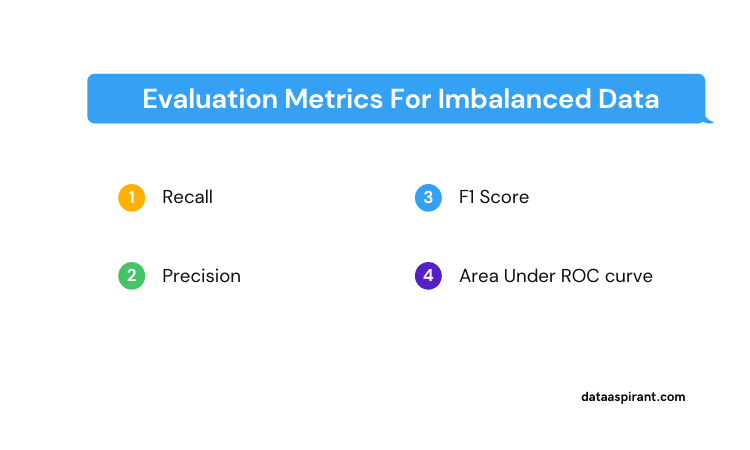Evaluation Metrics for imbalance data

We can use any of the below-mentioned metrics for unbalanced or skewed datasets.

• Recall
• Precision
• F1-score
• Area Under ROC curve.

We can see the huge difference among different evaluation metrics for both classifications (decision tree & random forest) models.

Do you remember we mentioned at model development stage, accuracy, classification report, etc. ?

Okay, let see the results here.

### Random Forest Classification model results

Here we have to discuss a few terms and formulae related to confusion matrix, precision, recall & F1-score.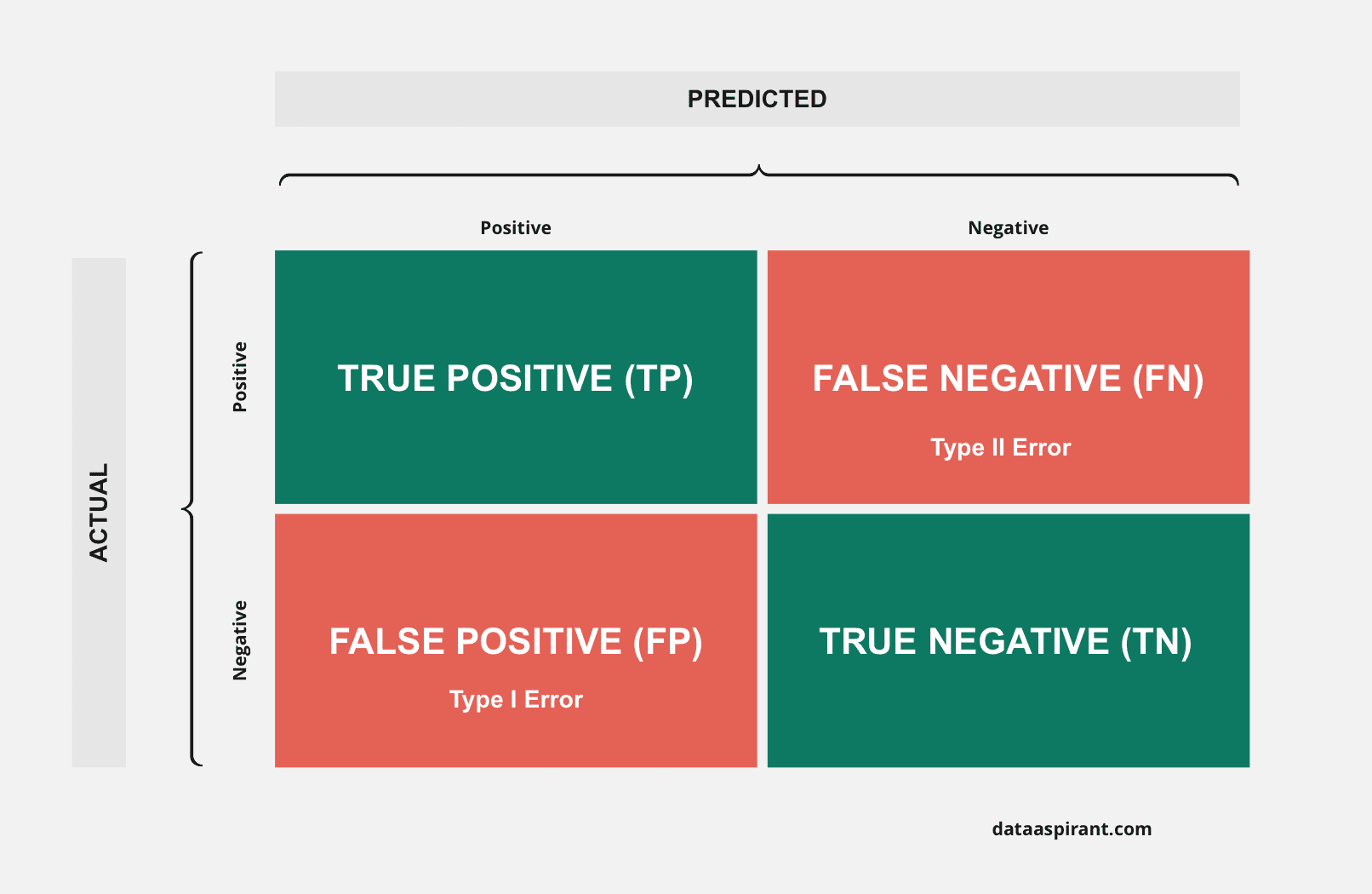1. True Positive (TP):-

The number of positive labels correctly predicted by trained models.  This means the number of Class-1 samples correctly predicted as Class-1.

1. True Negative (TN):-

The number of negative labels correctly predicted by trained models.  This means the number of Class-0 samples correctly predicted as Class-0.

1. False Positive (FP):-

The number of positive labels incorrectly predicted by trained models. This means the number of Class-1 samples incorrectly predicted as Class-0.

1. False Negative (FN):-

The number of negative labels incorrectly predicted by trained models.  This means the number of Class-0 samples incorrectly predicted as Class-1.

#### Formulae

• Recall = TP / (TP + FN)
• Precision = TP / (TP + FP)
• F1-Score = 2*P*R / (P + R) here P for Precision, R for Recall

Both classification models got accuracy scores as 99%.

But when we observe the result of the classification report of both classifiers, f1-score for Class-0 got 100%, but for Class-1, F1-scores are significantly less.

All these variations occur due to the unbalanced or skewed dataset.

Why f1-score for class-0 100%?

Because of the number of samples for class-0 (2 lakhs). The number of samples for Class-0 is very high than the Class-1 samples.

So what we need to do here is handle an unbalanced dataset. If you want to learn more about it, check the Best ways to handle unbalanced data in the machine learning article which explained various ways to handle the imbalanced data.

One more thing is left for discussion in this section, which is about areas under the ROC curve.

### AUC and ROC Curves

Area Under ROC curve is another evaluation metric for classification problems. This is mostly suitable for skewed datasets. It tells us about model performance, such as the model’s capability to distinguish between target classes.

The effective model has a higher Area Under the ROC curve value. Here we measure the ability of class separability of a model by using the Area Under ROC curve.

Good models have AUC value near to 1, and the worst models have AUC value near 0.

All the model performance methods help in the measuring the performance of the model based on the problem, but how to build the best models when we face with the data imbalance issue?

For that, we need to apply different sampling methods to the data before building the models.

Let’s see how sampling methods improve model performance, and how much AUC score for that model in the coming section.

## Model Improvement Using Sampling Techniques

Data sampling is the statistical method for selecting data points (here, the data point is a single row) from the whole dataset. In machine learning problems, there are many sampling techniques available.

Here we take undersampling and oversampling strategies for handling imbalanced data.

What is this undersampling and oversampling?

Let us take an example of a dataset that has nine samples.

• Six samples belong to class-0,
• Three samples belong to class-1

Oversampling = 6 class-0 samples x  2 times of class-1 samples of 3

Undersampling = 3 Class-1 samples x 3 samples from Class-0

Here what we are trying to do is the number of samples of both target classes to be equal.

In the oversampling technique, samples are repeated, and the dataset size is larger than the original dataset.

In the undersampling technique, samples are not repeated, and the dataset size is less than the original dataset.

### Applying Sampling Techniques

For undersampling techniques, we are checking the number of samples of both classes and selecting the smaller number and taking random samples from other class samples to create a new dataset.

The new dataset has an equal number of samples for both target classes.

This is a whole process of undersampling, and now we are going to implement this entire process using python.

The above is the target class distributions, now let’s see how we can change this.

Here first, we take indexes of both classes and randomly choose Class-0 samples indexes that are equal to the number of Class-1 samples.

In the below code snippet, Combine both classes indexes. Then we extract all features of gathered indexes.

The above code first divides features and targets as x_undersample_data and y_undersample_data and then splits new undersample data into train and test dataset.

Okay, now we will call both classifiers with these new under sampling train and test datasets.

### Decision tree classification after applying sampling techniques

Below are the model performance details

### Random Forest Tree Classifier after applying the sampling techniques

Below are the model performance details after applying the sampling techniques.

See, the results of the F1-score for both target values are 95%, and the Area Under ROC curve is near to 1.

For the best models, we have the AUROC value near to 1. Here we implemented the undersampling technique; you can apply oversampling also like an undersampling process.

## Conclusion

Finally, our model gives 94% of the Area Under the ROC curve value. We can improve model results by adding more trees or applying additional data preprocessing techniques, etc.

Not only decision trees or random forest classifiers suitable for this problem. You can try with other machine learning classification algorithms such as Support Vector Machines (SVM), k-nearest neighbors, etc.  to check how different algorithms are performed on classifying fraudulent activities.

## What next

Try to use different classification algorithms to solve the same problem and check the F1 score for all the models. For implementation, you can have a look at the code snippets from the below articles.

#### Recommended Courses#### Credit Risk modelling in Python#### Data Science Bootcamp Course